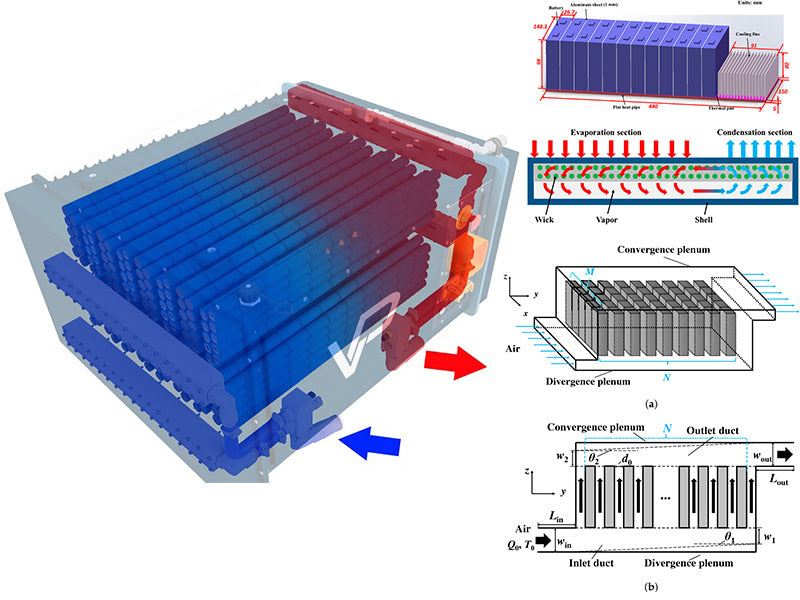• 1. Serial ventilation
• 2. Parallel ventilation
•

The temperature difference between different battery cells and battery modules in the battery box (or battery pack) will aggravate the inconsistency of the internal resistance and heat of the battery. If it accumulates for a long time, it will cause some batteries to be overcharged or overdischarged, which will affect the battery life and performance, and cause safety hazards. The temperature difference between different battery cells has a lot to do with the arrangement of the battery pack/module. Generally, the battery temperature in the center is high, and the temperature at the edge is low due to good heat dissipation conditions. Therefore, when arranging the battery pack/module structure and heat dissipation design, it is necessary to ensure the uniformity of cooling of each battery cell as much as possible. According to the ventilation mode, air-cooled battery cooling is mainly divided into two modes: serial ventilation and parallel ventilation. The basic principle is shown in Figure 1.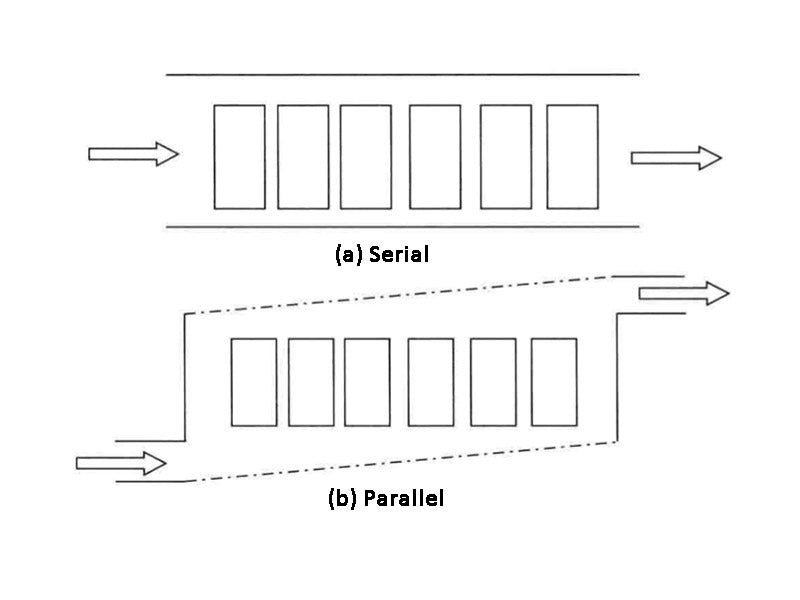Figure 1 - Schematic diagram of serial and parallel ventilation

Figure 1(a) shows serial ventilation, where low-temperature air enters from the left side and exits the battery module from the right side. The air is continuously heated during the flow, so the heat dissipation effect of the battery on the right is worse than that on the left, and the temperature of the battery on the right is likely to be higher than that on the left. Figure 1(b) shows parallel ventilation. Through the wedge-shaped air intake and exhaust channel design, the air flow between the battery cells and battery modules is made to pass in parallel, which is conducive to more uniform distribution of air among different battery cells and battery modules.

## 1. Serial ventilationHe et al at Virginia Tech constructed a serially ventilated battery module. The battery module consists of 8 A12326650 power batteries (2.3A·h, 3.3V) in 4 series and 2 rows in parallel. The final battery module voltage is 14.8V and the capacity is 4.6A·h. The experimental platform includes charging and discharging equipment, temperature, pressure, speed measurement devices, control devices, wind tunnel devices, etc. The wind tunnel device can effectively control the wind speed, and the wind speed range is 0.5~30m·s-1. He et al. carried out a two-dimensional numerical simulation study of this module with the help of ANSYS/FLUENT software. The numerical model ignores the changes of fluid parameters and the direction of the flow field. The schematic diagram of the CFD model and the meshing results are shown in Figures 2(a) and (b), respectively. The mesh adopts a quadrilateral unstructured mesh. Due to the relatively large velocity gradient near the battery, the boundary layer mesh has been refined.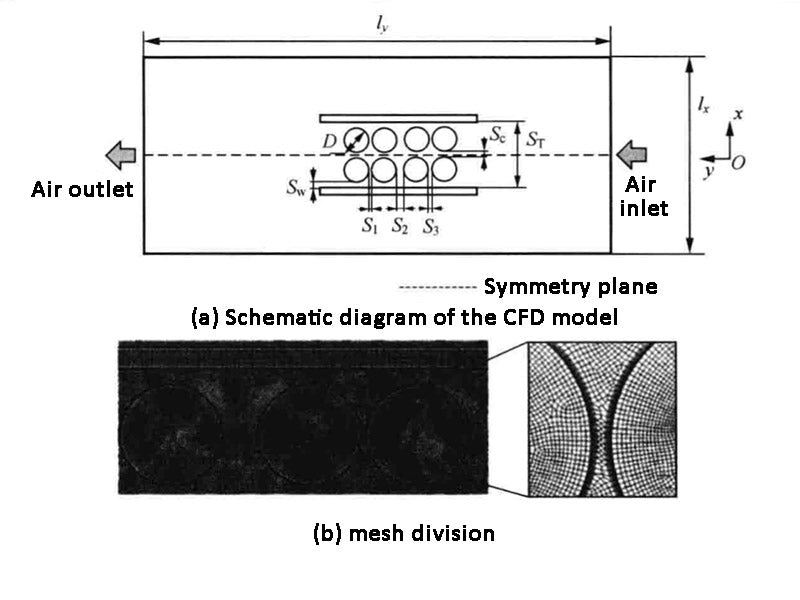Figure 2 - Schematic diagram of the CFD model and meshing

The research idea of H et al. is to use the experimental results to verify the numerical model, and then use the numerical model to study the experimental scheme that is inconvenient to measure or relatively expensive. In this serial ventilated battery pack model, He et al. obtained experimental measurements of temperature, air flow rate, and pressure representative of the battery module. A comparison of their experimental and simulated results agrees well. Afterwards, they used the validated numerical model to simulate and analyze the energy consumption under different wind speeds and different battery-to-case distances, and the results are shown in Figure 3. It can be seen from the figure that for the two spacing conditions, as the wind speed increases, the energy consumption power increases, and when the wind speed increases from 0.1m·s-1 to 10m·s-1, the energy consumption increases by 5 orders of magnitude, which shows that optimizing the energy consumption of the battery thermal management system is important. The energy consumption when the battery case spacing is 5mm is greater than that when the spacing is 17mm. This is because at the same wind speed, the spacing increases, the (Re) Reynolds number decreases, and the friction coefficient decreases.Figure 3 - Energy consumption at different wind speeds and distances

## 2. Parallel ventilation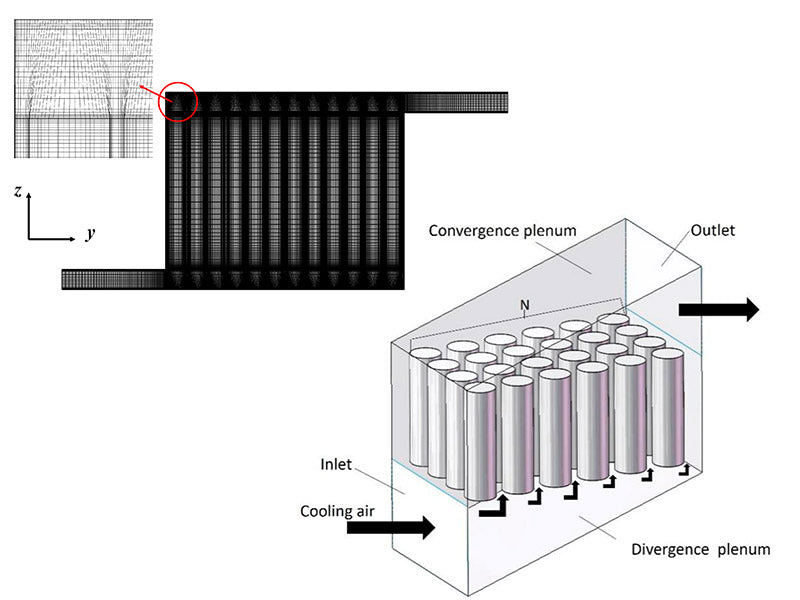Liu et al. of Tianjin University constructed a simplified calculation model of parallel ventilation to predict the air flow velocity and temperature distribution of the battery pack in large air-cooled battery packs. The schematic diagram of this simplified calculation model of parallel ventilation is shown in Figure 4. Figure 4(a) shows the entire large battery pack, which consists of wedge-shaped inlet and outlet air ducts and several identical battery modules. The structure of the battery module is shown in Fig. 4(b). The battery module consists of 8 battery cells containing 5 battery cells, and the single cells are cylindrical 18650 cells. Two adjacent battery cells in the same battery unit are connected by a plate, so that a separate cooling channel is sandwiched between the two groups of battery cells. Parameters such as the angle θ of the air duct, the minimum width ωmin at the end of the air duct, and the distance between the battery cells lsp are the research objects that affect the speed and temperature distribution.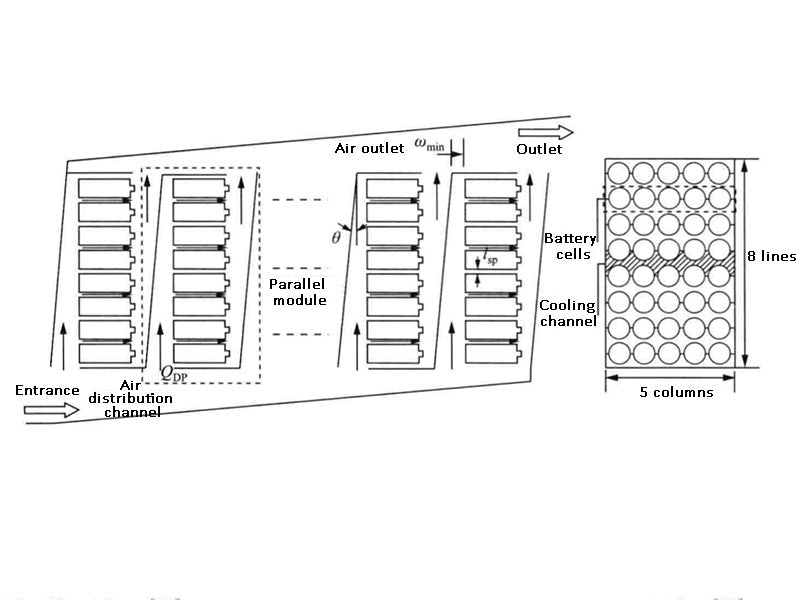Figure 4 - Schematic diagram of the parallel ventilation model

Such simplified computational models include flow resistance network models and transient heat transfer models. The flow resistance network model mainly calculates the irreversible pressure loss, including local loss and friction loss, and then obtains the velocity distribution of the entire system through the transformation relationship between pressure and velocity; the transient heat transfer model uses the velocity field derived from the flow resistance network model to calculate the convective heat transfer coefficient, and then combine the heat production obtained by the battery heat production model and the battery cell centralized parameter thermal model to obtain the temperature changes of the battery cells, battery cells and battery modules. Liu et al. used the Runge-Kutta algorithm to discretize the relevant differential equations in the transient heat transfer model, and solved the flow resistance network model and the transient heat transfer model through MATLAB software programming. The calculation results of this simplified calculation model are compared with the results of the CFD model, and the two are in good agreement.

Figure 5(a)~(c) respectively reflect the influence of the angle θ of the air duct, the minimum width ωmin at the end of the return air duct, and the cell spacing lsp on the uniformity of the speed and temperature distribution of the battery cells. It can be seen from the figure that the uniformity of speed and temperature increases with the increase of the angle θ of the air duct. In order to make the maximum temperature difference of the battery cells less than 5°C, when discharging at 5C, the angle θ cannot be less than 16.5°; during 4C discharge, the angle θ cannot be less than 13.2°. Similarly, when the angle θ is 10°, the maximum temperature difference during discharge at 5C and 4C can be less than 5°C only when the spacing lsp of the battery cells is at least 16.4mm and 10.1mm, respectively. Increasing the two parameters of θ and lsp can reduce the pressure loss of the flow channel, but it also significantly increases the volume of the battery module and reduces the energy density of the battery module. When θ is 10° and lsp is 1mm, the minimum width Wmin at the end of the return air duct is at least 14.1mm and 8.3mm, respectively, to satisfy the maximum temperature difference between 5C and 4C when the discharge is less than 5°C. The comparison results show that only adjusting the size of the return air duct ωmin is more advantageous than adjusting the inlet air duct and the return air duct ωmin at the same time, because the pressure drop of the inlet air duct is not as obvious as that of the return air duct. Figure 5(d) shows the inlet flow, maximum temperature and minimum temperature of each battery module in the battery pack. It can be seen from the figure that the volume average temperature of each module is not equal and is inversely correlated with the inlet flow.Figure 5 - Air-cooled heat dissipation characteristics

Heesung of South Korea's Hyundai Motor Company designed a special forced air cooling heat dissipation model according to the thermal performance requirements, and studied different ventilation structures by combining theoretical analysis and numerical simulation, and then carried out structural optimization design. The system consists of two rows of battery packs, each consisting of 36 battery cells and 37 cooling channels. The voltage of the entire battery pack is 270V, the energy storage is 1400W·h, and the surface heat flux density of the single cell is 245W·m-2.

The cooling channel flow distribution directly affects the temperature uniformity of the battery pack, and the channel structure is crucial to the flow distribution. On this premise, Park designed five different ventilation structures, as shown in Figure 6(a)~(e). The intake manifold and return air manifold of the first solution are rectangular, and the intake and return air manifolds of the second and third solutions are angled respectively, the fourth scheme changes the direction of the outlet to the reverse on the basis of the third scheme, and the fifth scheme opens a narrow long hole on the right side on the basis of the third scheme.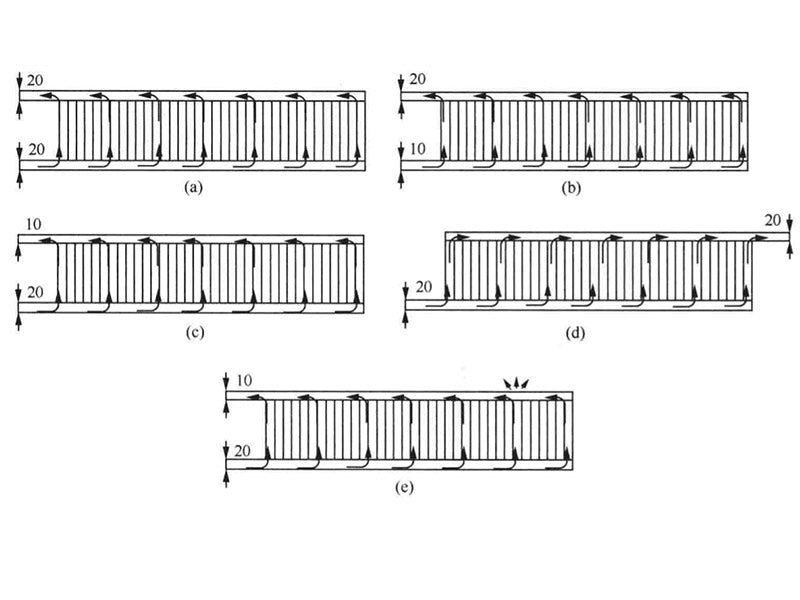Figure 6 - Five different ventilation methods

Figure 7(a)~(e) reflect the temperature distribution cloud diagrams of the battery packs with five different ventilation structures. As can be seen from the graph, the maximum temperature of the battery pack is directly affected by the flow through the cooling channels. The highest temperature in the first way occurs on the battery farthest from the air outlet, due to the smallest air flow in the runner on the far right. The second method of gradually releasing the population and tapering the outlet makes the location of the highest temperature of the battery pack move towards the direction of the air outlet, and the heat transfer deteriorates, and the highest temperature is much higher than that of the first method. The third method of tapered inlet and outlet can greatly reduce the maximum temperature, but it still cannot meet the thermal design requirements. For the case where the inlet and outlet are on the same side, due to the sharp increase in the flow channel pressure at the population outlet, the flow rate is greatly reduced, and the heat transfer performance deteriorates, resulting in a large temperature difference in the battery pack. The fourth method can greatly reduce the maximum temperature. In this way, it can be seen that releasing the pressure of the channel far away from the inlet can greatly improve the uniformity of the flow distribution, thereby improving the cooling performance. The fifth method draws on the third and fourth methods, the maximum temperature is lower than the fourth method, and the maximum temperature difference is lower than the design requirements of 20℃.Figure 7 - Cloud map of temperature distribution under five ventilation modes